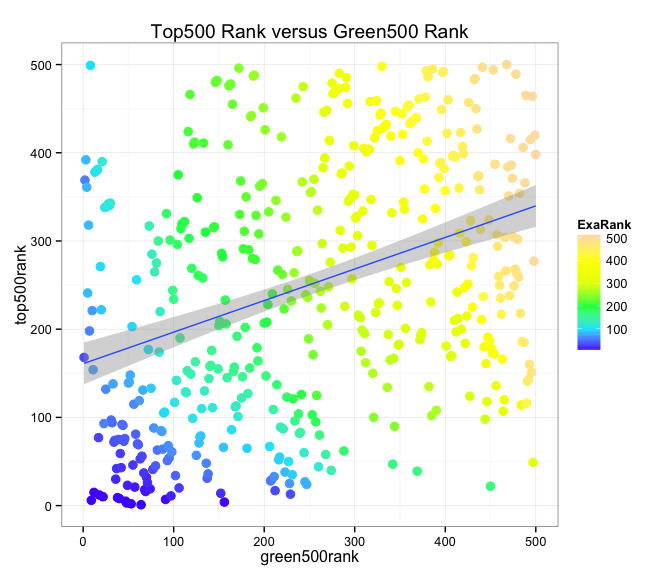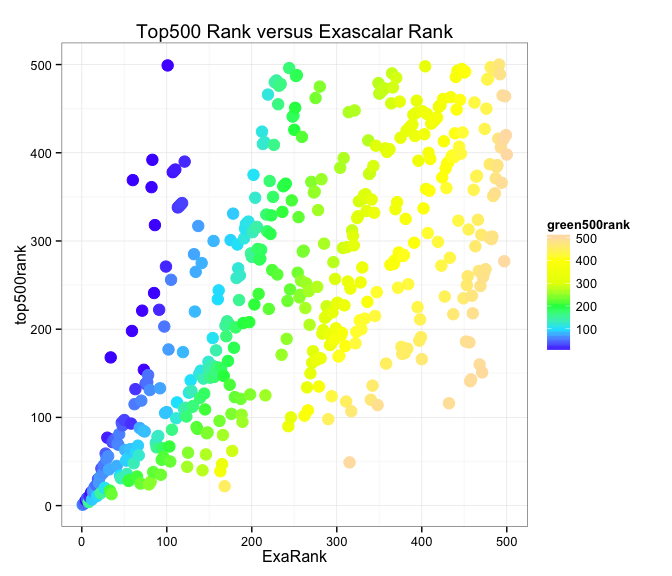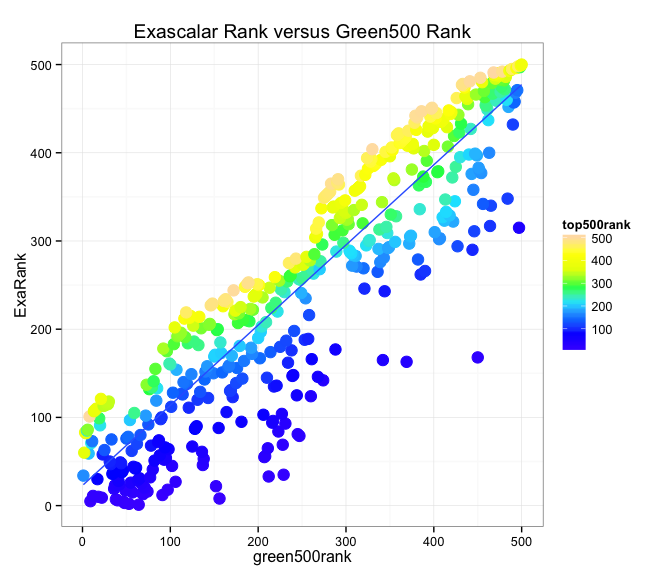Looking for correlations Top500, Green500, and Exascalar

Exascalar is one of the best ways to visualize changes in the Green500 and Top500 lists since it compares system performance and efficiency in one common analysis to revela trend in the population.

Exascalar (and its orthogonal complement, power) are prefered for describing population trends as they closely correspond to the principal components of an idealized “Triangular” Exascalar distribution. Exascalar and Power best model the observed variance of the distribution population.

In this study we’ll explore these correlations and show that while the Top500 lists and Green500 lists appear uncorrelated, Exascalar can serve as a lens through which correlation can be understood.

Top500 and Green500 data appear uncorrelated

Although it is intuitively obvious that a higher efficiency ranking should be related to higher performance ranking, a straight plot of the Top500 and Green500 shows little correlation between the two lists.

Data points have been color coded to reflect the Exascalar ranking. Note that the Exascalar ranking creates kind of “bands” linking the two scales.A linear fit shows that the green500 predicts only 12.8 % of the variation in the Top500.

Using Exascalar as a predictor of Top500 rank

A very different picture emerges, however, if we use Exascalar as a predictor of Top500 rank and factor the data for the Green500 ranking. The correlation of Exascalar to performance is well known, so this observation is not a surprise.Green500 as a predictor of Exascalar Rank

The graph below shows the Green500 rank as predicted by the Exascalar rank. Here a clear correlation is again observed, with high exascalar rank (low rank number) predicting a high Green500 rank (low rank number).

It might be surprising that a low Top500 ranking (again a low ranking corresponds to a high rank number) should translate to a higher Green500 ranking (lower rank number). This counterintuitive result comes about because of the “negative” slope of the Exascalar line. Recall that for Exascalar an increase in performance at constant efficiency(through higher power) is valued the same as an increase in efficiency at constant performance.A linear fit of the data predicts about 82.6% of the observed variation.

Some conclusions

Top500 and Green500 lists measure a common set of computers, yet their ranking appears to be largely uncorrelated. However, perhaps counter intuitively, we can use Exascalar, which aligns to the mathematically defined principal component of the distribution, as a kind of lens to understand how Green500 rank -> Exscalar rank -> Top500 rank.

This works at least partically because implicit counfounding effects (e.g. a dependence on power in the case of performance = efficiency * power) is comprehended through the “lens” of Exascalar.

Exascalar does not replace the necessary lists of the Top500 and Green500 lists, but it does complement them by providing a mutual context for linking the two lists and describing the variation of the population of supercomputers.